Published

# IoT Coin Counter

This revolutionary coin counter is used to make the penny wars donation drive more efficient with an online dashboard

IntermediateShowcase (no instructions)1,772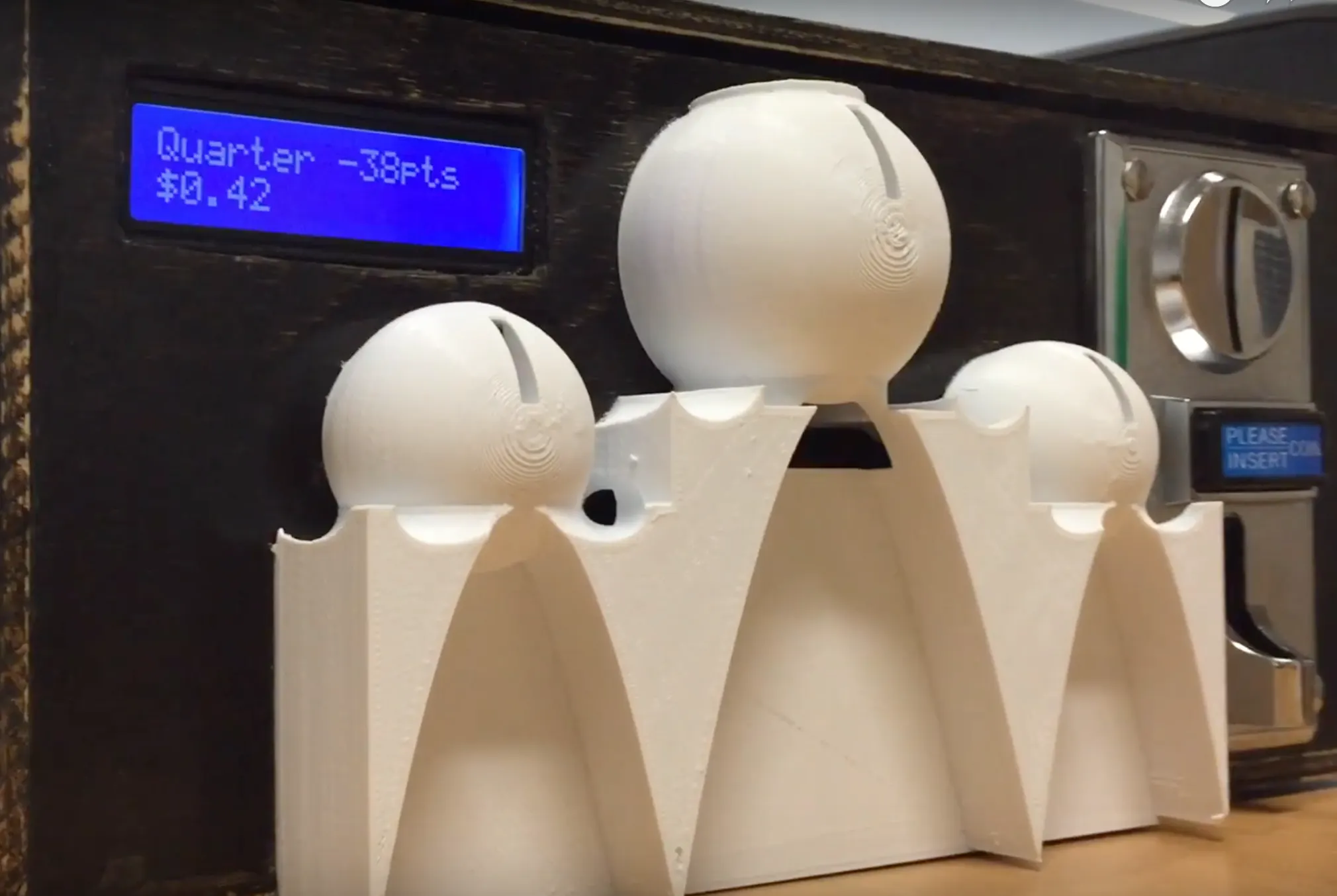## Things used in this project

### Hardware components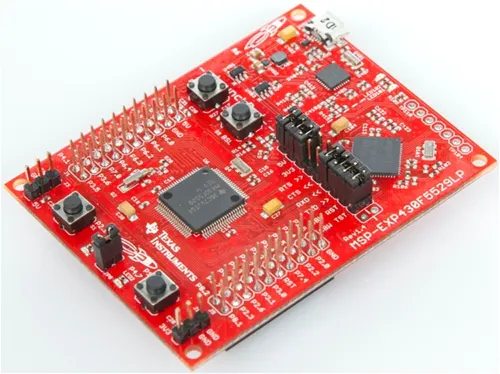×1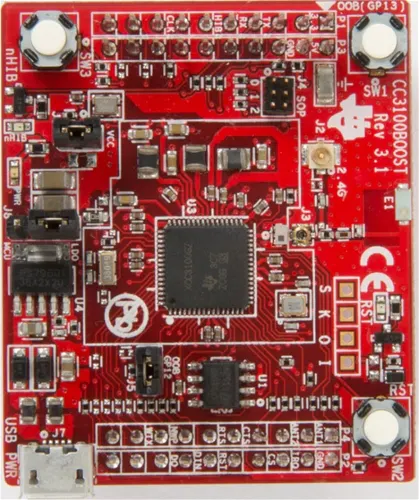Texas Instruments CC3100BOOST SimpleLink CC3100 Wi-Fi BoosterPack
×1Jumper wires (generic)
×1
×1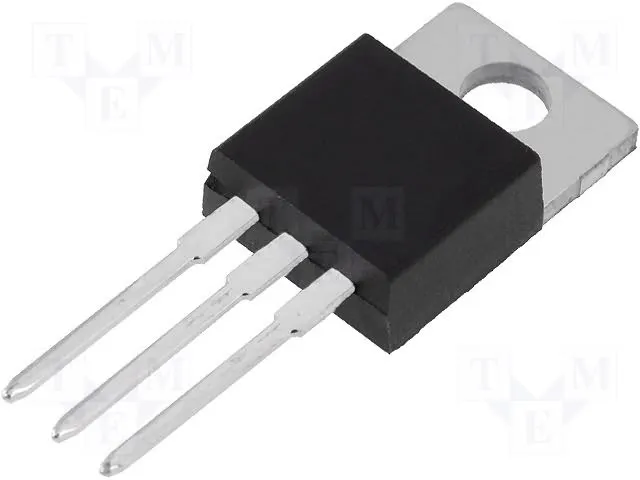Linear Regulator (7805)
×1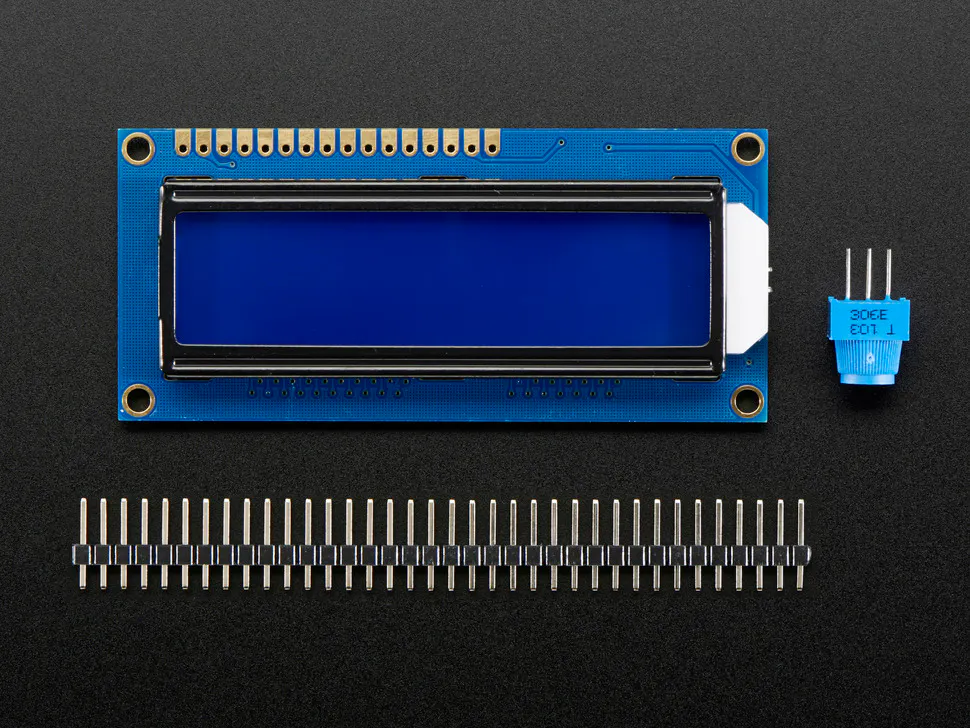Adafruit Standard LCD - 16x2 White on Blue
×1×1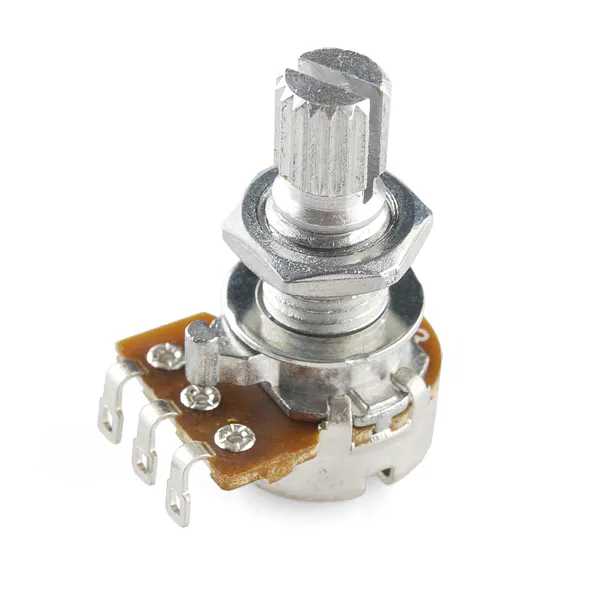Rotary potentiometer (generic)
×1

### Software apps and online servicesIBM BlueMixTexas Instruments Energia

## Schematics

### Block Diagram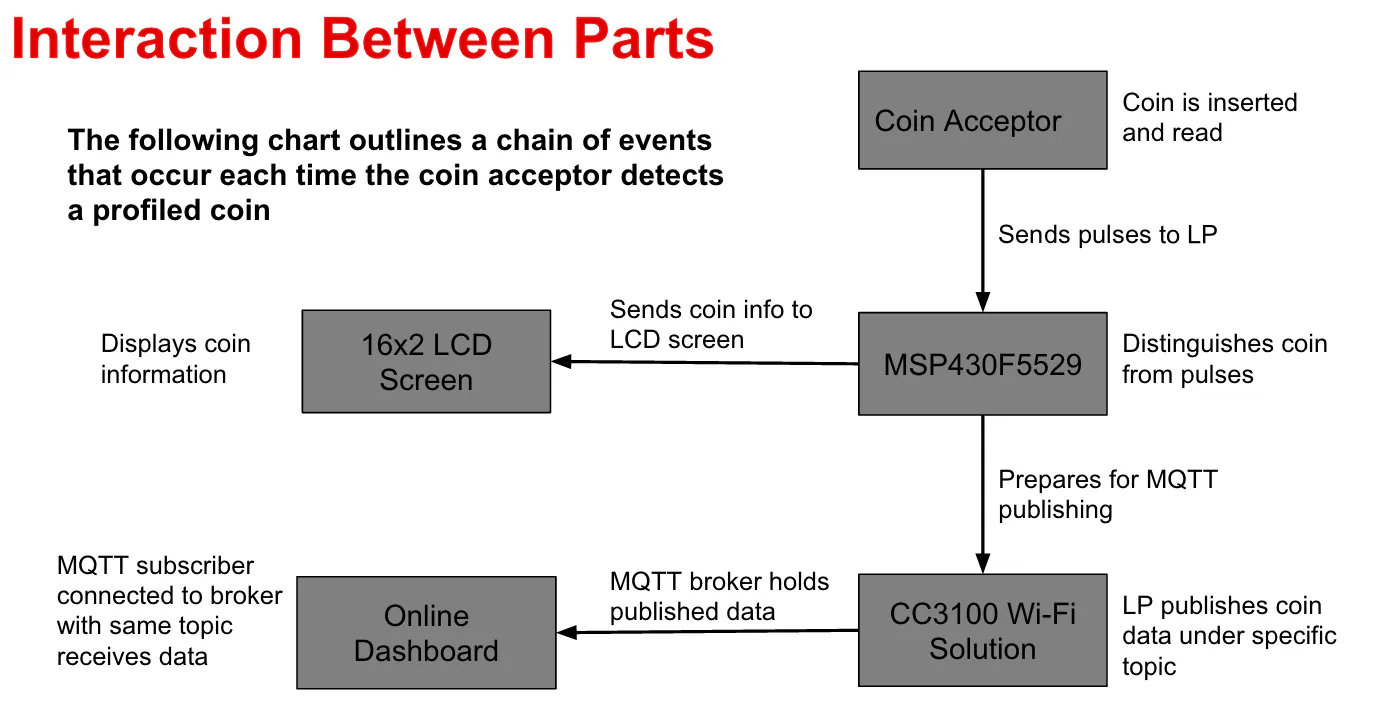## Code

### IoT Coin Counter Code

C/C++
```#include <WiFi.h>
#include <PubSubClient.h>
#include <SPI.h> //only required if using an MCU LaunchPad + CC3100 BoosterPack. Not needed for CC3200 LaunchPad
WiFiClient wclient;

byte server[] = { 198, 41, 30, 241 }; //  Public MQTT Brokers: http://mqtt.org/wiki/doku.php/public_brokers
byte ip[]     = { 172, 16, 0, 100 };

#define       WIFI_SSID         "WiFi Name"//input wifi name in quotes

PubSubClient client(server, 1883, callback, wclient);

void callback(char* inTopic, byte* payload, unsigned int length){
// Handle callback here
}

#include <LiquidCrystal.h>

LiquidCrystal lcd(23, 24, 25, 26, 27, 28, 31, 32, 11, 12);

const short buttonPenny= 9;
const short buttonNickel= 10;
const short buttonDime= 29;
const short buttonQuarter= 30;

short pennyButtonState = 0;
short nickelButtonState = 0;
short dimeButtonState = 0;
short quarterButtonState = 0;

unsigned  int numPennies = 0;
unsigned  int numNickels= 0;
unsigned  int numDimes= 0;
unsigned  int numQuarters = 0;
double totalValue=0;
int points= 0;

const int coinInt = 39;

int pulseCount = 0;
unsigned long time;
unsigned long current = 0;

void setup()
{
lcd.begin (16, 2);
Serial.begin(9600);

pinMode (buttonPenny, INPUT_PULLUP );
pinMode (buttonNickel, INPUT_PULLUP );
pinMode (buttonDime, INPUT_PULLUP );
pinMode (buttonQuarter, INPUT_PULLUP );

Serial.println("Start WiFi");
WiFi.begin(WIFI_SSID, WIFI_PWD);
Serial.print(".");
delay(300);
}
Serial.println("");

printWifiStatus();

attachInterrupt(coinInt, coinInserted, RISING);

}

void coinInserted()
{
current = millis();
pulseCount++;
}

void loop()
{

time = millis();
lcd.setCursor  (0, 0);

//time = millis();
if (current != 0)
{
if (time - current > 130) {

if (pulseCount == 1 ){
lcd.clear();
numPennies++;
lcd.print("Penny");
//delay (200);
}

else if (pulseCount == 2){
lcd.clear();
numNickels++;
lcd.print("Nickel");
//delay (200);
}

else if (pulseCount == 3){
lcd.clear();
numDimes++;
lcd.print("Dime");
//delay (200);
}

else if (pulseCount == 4){
lcd.clear();
numQuarters++;
lcd.print("Quarter");
//delay (200);
}

current = 0;
pulseCount = 0;

}
}

totalValue =  numPennies*0.01 + numNickels*0.05 +
numDimes*0.1 + numQuarters*0.25;

points=numPennies - (numNickels*5) - (numDimes*10) - (numQuarters*25);

lcd.setCursor  (0,1);
lcd.print ("\$");
lcd.print (totalValue);
lcd.setCursor  (8,0);
lcd.print(points);
lcd.print("pts");

String total = (String) totalValue;
int pos = 0;
int len = total.length();
while ((pos < len-1) && (total.charAt(pos) == '0'))
pos++;
total = total.substring(pos);

String str = (String) total;

if(totalValue< 1){
str = (String)"0" + total;
}

int str_len = str.length() +1;  // Length (with one extra character for the null terminator)
char char_array[str_len];  // Prepare the character array (the buffer)
str.toCharArray(char_array, str_len);  // Copy it over

String str1 = (String) points + " pts";
int str1_len = str1.length() +1;  // Length (with one extra character for the null terminator)
char char_array1[str1_len];  // Prepare the character array (the buffer)
str1.toCharArray(char_array1, str1_len);  // Copy it over

if (current != 0)
{
if (time - current > 130) {

if (pulseCount == 1 ||  pulseCount == 2||
pulseCount == 3 || pulseCount == 4)
{
Serial.print("\$");
Serial.println (totalValue);
Serial.print(points );

if(points ==1)
{
Serial.println(" Point");
}

else
{
Serial.println(" Points");
}

client.publish ("topicOne", char_array);//input topic desired
client.publish ("topicTwo", char_array1);//input second topic desired
Serial.println("Publishing successful!\n"  );
client.disconnect ();
}

}
}
}

}

void printWifiStatus() {
// print the SSID of the network you're attached to:
Serial.print("SSID: ");
Serial.println(WiFi.SSID());

Serial.println(ip);

}
```

## Credits

### Ali Soomar

11 projects • 37 followers
Student at the University of Texas at Austin

### Jeremy Tanueco

2 projects • 2 followers

### Tiffani Weir

2 projects • 1 follower